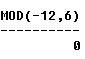## SQL MOD Function

SQL MOD function returns the remainder value which is a result of division of two numbers.

The syntax is

MOD(number, divider)

Examples:
The following SQL statements illustrate the use of SQL MOD function on couple different numbers:

SELECT MOD (34, 6) FROM DUAL;

OUTPUT:SELECT MOD (24, 4) FROM DUAL;

OUTPUT:SELECT MOD (4,12) FROM DUAL;

OUTPUT:SELECT MOD (-12,6) FROM DUAL;

OUTPUT: